Browse by Topic
Related Topics

# Wolfram SystemModeler in Electrical Engineering Courses

Explore the contents of this article with a free Wolfram SystemModeler trial. I’m an electrical engineer by training. In my first circuits class, all calculations were done by hand, and we could check solutions with unintuitive circuit simulators using the SPICE methodology. With SystemModeler I think it’s easier than ever to get started building virtual circuits and trying what-if scenarios for electrical circuits and systems. In this blog post, I’ll start from very basic circuits with components such as resistors and inductors and gradually add more complexity in the form of amplifiers and switching circuits.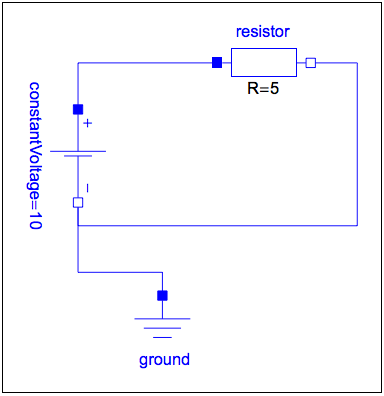I named it HelloSystemModeler in the tradition of computer language tutorials, where most first simple programs usually start with a greeting like “Hello, world.” This circuit only contains three components: a resistor, a voltage source (like an ideal battery), and a ground. To create this circuit, I used drag-and-drop from the built-in components that come with SystemModeler.

This circuit is something that you would build in middle school (at least I did), with the resistor replaced with a small light bulb. You’d also learn Ohm’s law, stating that I = V/R, so with a potential of 10 volts and a resistance of 5 ohms, we get a current of 2 amperes. Let’s see how SystemModeler handles this.

Simulate the circuit using WSMSimulate: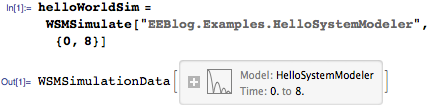I can now query the simulation object for all variables and parameters. Let’s look at the current in the resistor: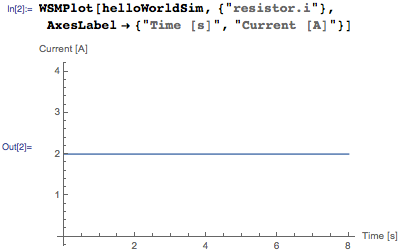Now let’s go for something a bit more interesting and usable, say, an RC circuit. The name refers to the two components in the circuit: a resistor (R) and a capacitor (C). Together they act as a low-pass filter that will filter out the high-frequency components of a signal.The capacitor and resistor components are defined directly from their constitutive equations: for a capacitor, we in general have the equation I(t) = C dV(t)/dt, and if we look at the model for the capacitor, we see exactly this equation popping up: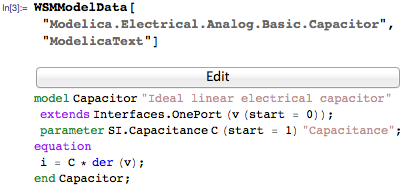The code above is written in the object-oriented modeling language Modelica, which SystemModeler implements. Not shown here is the annotation in the code that specifies how the component should look when used in the graphical user interface.

The same way of defining components from equations works for the resistor, inductor, and so on. This brings a very powerful concept to the modeling table, namely, to be able to define components and let the computer figure out a form of the complete system that can be simulated. Less burden on the modeler and more work for the computer. If I didn’t have such an acausal modeling environment, I’d have to derive the complete relationship from input to output, and then implement that function. That would look something like this: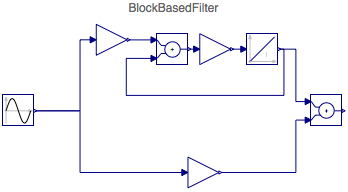Not only has the real-world reassemblance completely disappeared, but it’s also hard to reuse this if I want to change the structure and add an extra component, say an inductor, to it. I would have to redo all my work, whereas in SystemModeler I can simply connect it where I want it, and the system will regenerate the simulation equations.

Returning to the RC circuit, in the diagram below, the filter is connected to the power sources and a load resistor.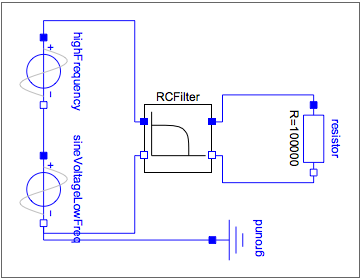Next I run a simulation with this model and can directly see the result of the filter. In the diagram below, the high-frequency signal is damped.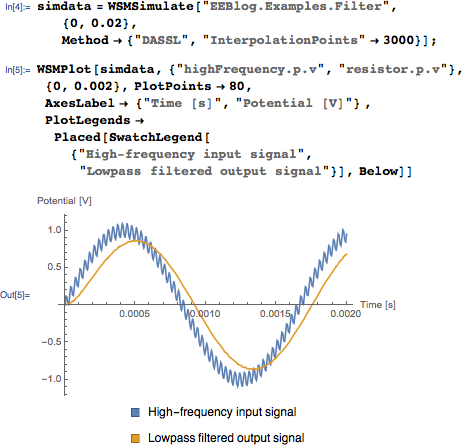Another way to see this is to look at the frequency spectrum of these signals. First I sample the signals: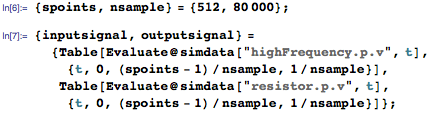Then I can look at their spectra by using the discrete Fourier transform: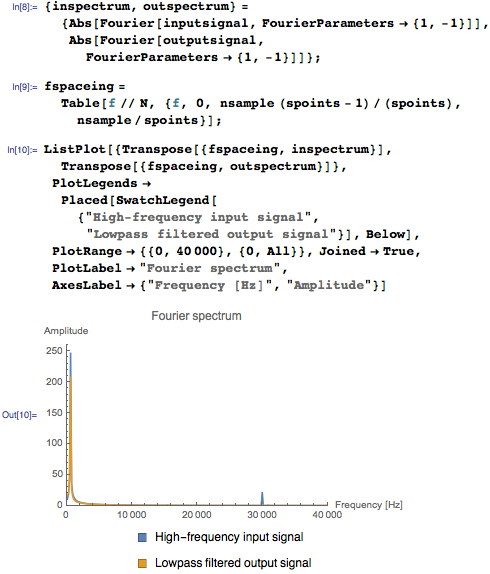Here I can see the peak 600 Hz for both signals, and for the input signals there’s a second peak at 30,000 Hz, where the noise is added.

There are many ways to improve this filter, and one of them is to add an amplifier to the circuit. Let’s take a look first at an inverting amplifier circuit, which happens to be one of the first lab exercises I did at university.

The operational amplifier in the center of the next figure will amplify the voltage at the negative pin by a factor of resistor1/resistor = 30000/10000 = 3, if that value is within the limits from the two constant voltages I’ve connected to the circuit. If it’s outside the limits, the limits will be the value. The constant voltages are25 volts in this case.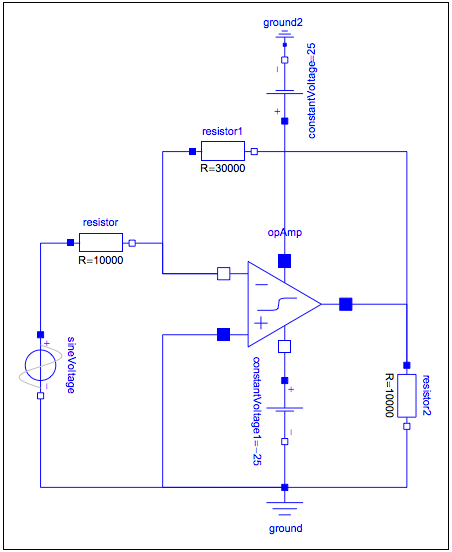Simulating this circuit, we can see exactly how that plays out: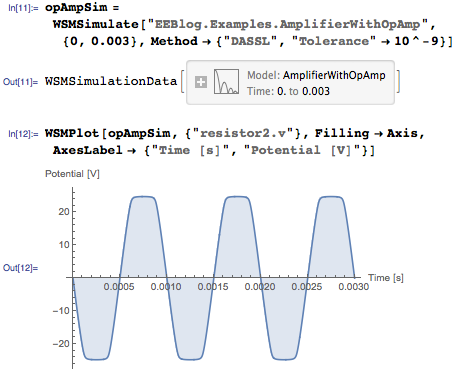The voltage reaches25 V, where the limit for the operational amplifier is, and saturates there.

There are many applications for operational amplifiers, such as amplifiers, regulators, and active filters. Let’s now look at an active low-pass filter, similar to our first RC filter, but with the operational amplifier added: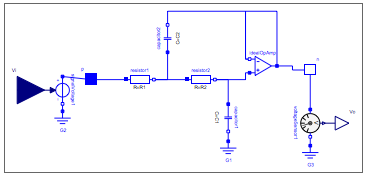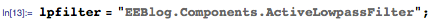An interesting application made possible by SystemModeler and Mathematica being symbolic tools is the possibility to derive analytical expressions for the transfer functions of circuits. To do that I’ll first get a linearized state-space model: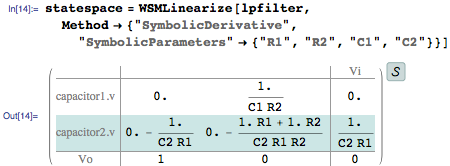The state-space model is a set of ordinary differential equations (ODEs) that relate the input Vi to the output Vo. The variables of the ODEs are capacitor1.v and capacitor2.v, which are the states of the state-space model.

It’s possible to directly convert the state-space model to a transfer function, displaying the relationship between input voltage Vi and output voltage Vo: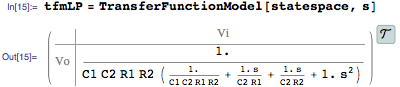Or display its Bode plot to show the cut-off frequency: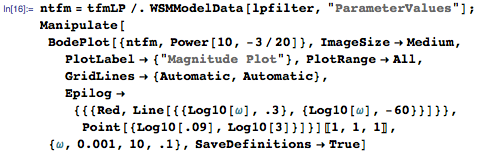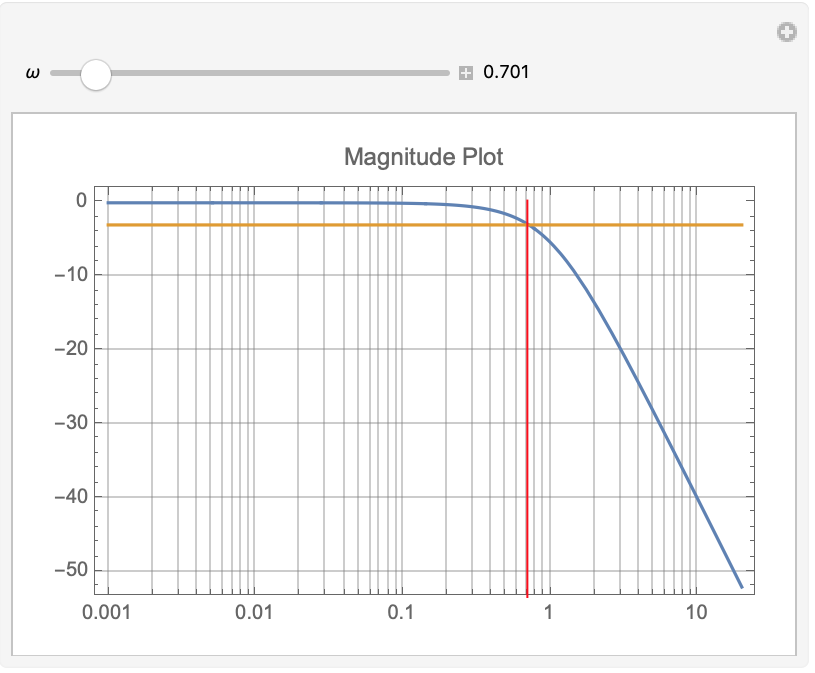The cut-off frequency is where the magnitude of output voltage is 3 dB lower than the input voltage. In the above plot, one can see that the frequency is around .7 rad/s by moving the slider.

So far, so good.

Now I’m going to look at an example where I don’t know how to directly apply Ohm’s law. Rectification is the process of taking an alternating current and turning it into a direct current. This is what happens in your laptop charger, which takes the AC supplied from the wall socket and converts it into DC that is usable in your computer.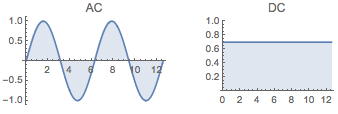To do that, a full-wave rectifier is usually used, with a so-called diode bridge: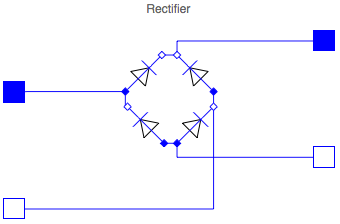The diodes in the bridge will “fire” so that a positive current will always flow into the positive pin on the DC side.

Next I connect this to a power source and a load: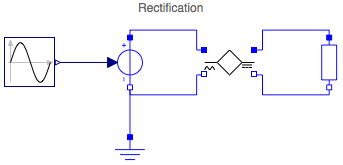Running a simulation, we can now see the how this full-wave rectifier converts the alternating current (blue) into a direct current (red):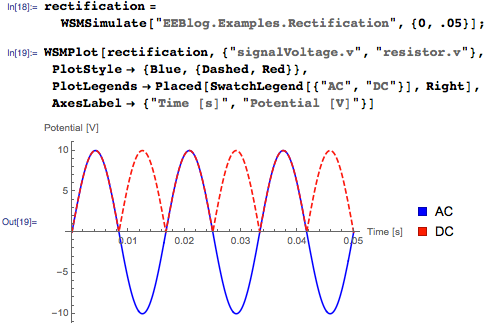As can be seen, there are a lot of ripples in this circuit. To smooth it out, a capacitor can be applied across the DC bus, like so: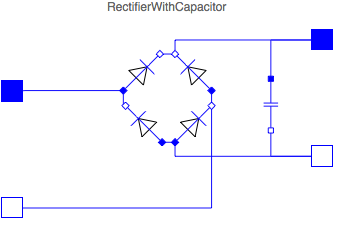Simulating with three different values for the capacitance of the capacitor, we get: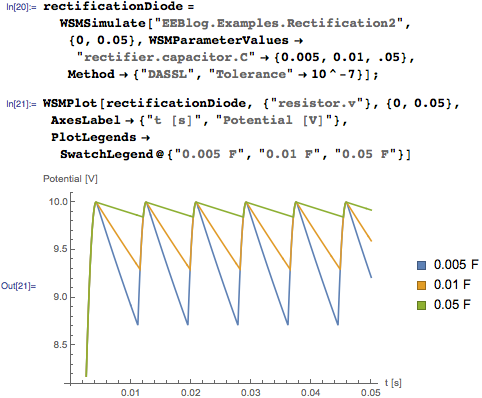I’ll pick one of these capacitor values, say 10 mF, and save the corresponding simulation in a separate variable: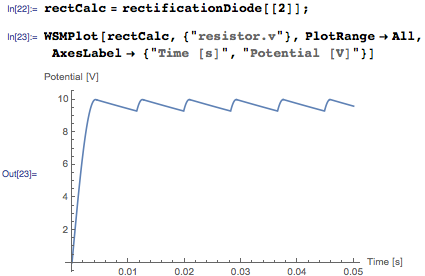And as we can see, the behavior is smoother. How much ripple is there? Instead of looking at values in the plot and calculating it with my calculator, Mathematica can do the work for me by finding the maximum and minimum values in a cycle: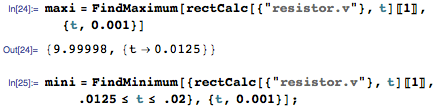Now that I have them, I can go on to mark them in a plot: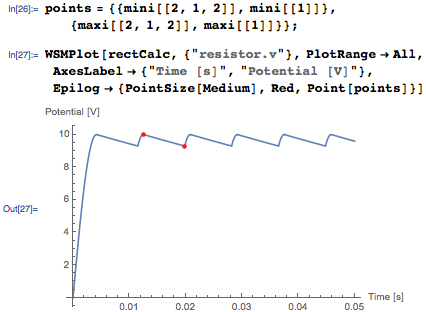Or just calculate the ripple as a percentage of the mean signal value: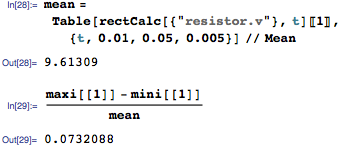OK, so that’s about a 7% ripple. We can improve this by adding an inductor on the DC bus side together with the capacitor.

Another interesting analysis task is to look at the efficiency of the conversion. For that task, I connect power sensors to the AC and DC sides.In a separate simulation, I integrate the power consumed on both sides and look at the quotient: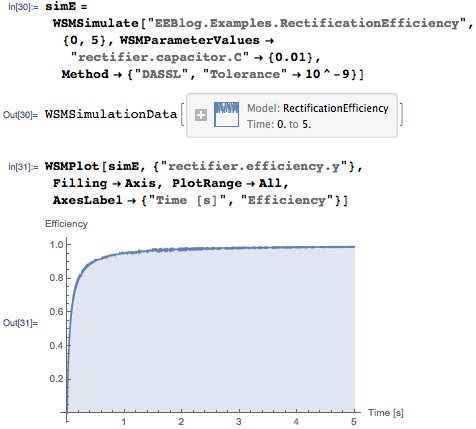As we can see, the efficiency at first is low, when the capacitor is charging. But later the efficiency is very high.

I’ll end this blog post by showing how these electrical components in turn can be connected to other domains. One such example is an electrical motor. So what I’ll do is connect our rectifier to a DC motor and some mechanical components, including a torque opposite of the motor’s that kicks in 10 seconds after the motor starts.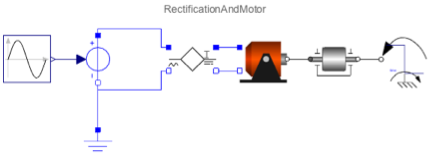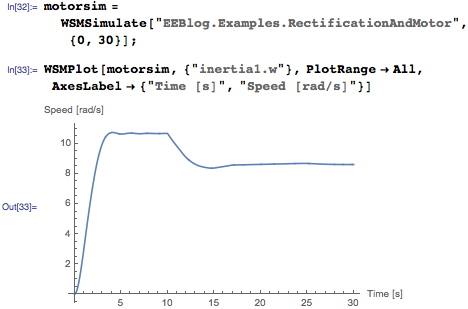The load connected to the motor will spin up and find a steady state value around 10 rad/s. After 10 seconds, a torque (the component on the right in the above diagram) with the opposite direction from the DC motor’s torque starts to turn, and the load is slowed down.

With the new release of SystemModeler 4, even more use cases are possible, including using the digital library to construct AD/DA converters and other digital circuits or quasi-stationary circuits for fast large analog circuit simulations. You can also create models directly from equations in Mathematica.

SystemModeler can give you a leg up in your next course or project. Keep an eye on the Wolfram Blog for further posts in this series.

Download this post, as a Computable Document Format (CDF) file, and its accompanying models.